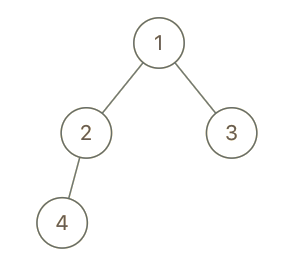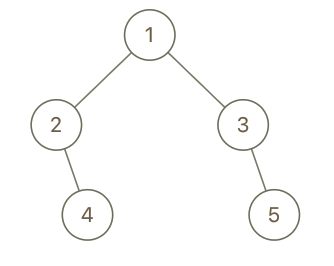# 993. Cousins in Binary Tree

Easy
Given the `root` of a binary tree with unique values and the values of two different nodes of the tree `x` and `y`, return `true` if the nodes corresponding to the values `x` and `y` in the tree are cousins, or `false` otherwise.
Two nodes of a binary tree are cousins if they have the same depth with different parents.
Note that in a binary tree, the root node is at the depth `0`, and children of each depth `k` node are at the depth `k + 1`.
Example 1:Input: root = [1,2,3,4], x = 4, y = 3
Output:
false
Example 2:Input: root = [1,2,3,null,4,null,5], x = 5, y = 4
Output:
true
Example 3:Input: root = [1,2,3,null,4], x = 2, y = 3
Output:
false
Constraints:
• The number of nodes in the tree is in the range `[2, 100]`.
• `1 <= Node.val <= 100`
• Each node has a unique value.
• `x != y`
• `x` and `y` are exist in the tree.

### 解題

Runtime: 0 ms, faster than 100%
Memory Usage: 2.5 MB, less than 82.86%
/**
* Definition for a binary tree node.
* type TreeNode struct {
* Val int
* Left *TreeNode
* Right *TreeNode
* }
*/
func isCousins(root *TreeNode, x int, y int) bool {
if root == nil {
return false
}
xLength := -1
xPre := root
yLength := -1
yPre := root
var findDepth func(*TreeNode, *TreeNode, int)
findDepth = func(root *TreeNode, pre *TreeNode, depth int) {
if root == nil {
return
}
if root.Val == x {
xLength = depth
xPre = pre
}
if root.Val == y {
yLength = depth
yPre = pre
}
findDepth(root.Left,root ,depth + 1)
findDepth(root.Right,root ,depth + 1)
}
findDepth(root, nil, 0)
return xLength == yLength && xPre != yPre
}